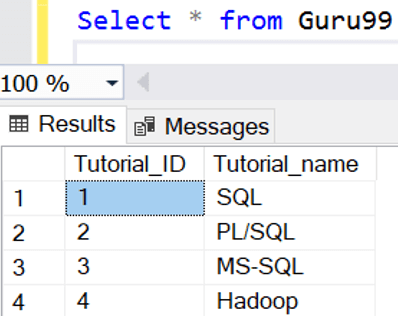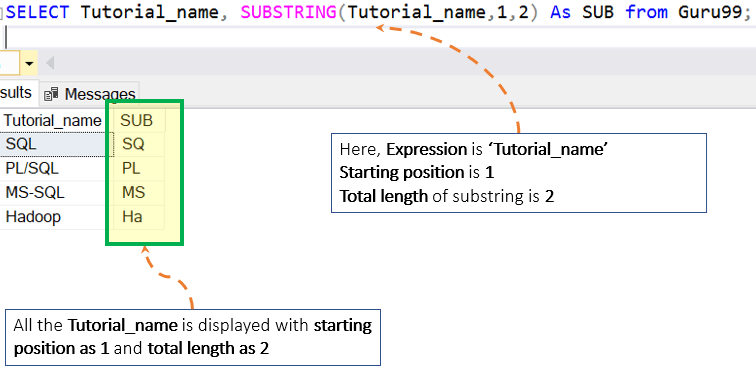# SQL Server SUBSTRING() Function: T-SQL Example

## What is SUBSTRING?

SUBSTRING is a function in T-SQL which allows the user to derive substring from any given string set as per user need.

### Syntax for substring

```SUBSTRING (Expression, Starting Position, Total Length)
```

Here,

• The Expression can be any character, binary, text or image. Expression is the source string of which we will fetch substring as per our need.
• Starting Position determines the position in expression from where the new substring should start.
• Total Length is the total expected length of result substring from the expression, starting from Starting Position.

### Rules for using SUBSTRING:

• All three arguments are mandatory.
• If the Starting Position is greater than the maximum number of character in Expression, then nothing is returned.
• Total length can exceed the maximum character length of the original string. In this case, resultant substring will be the entire string starting from Starting Position in expression till end character of Expression.

Below Diagram illustrate the use of SUBSTRING function.

### T-SQ Substring Examples:

Assumption: Assume that we have the table as ‘Guru99’ with two columns and four rows as displayed below:We will use ‘Guru99’ table in further examples

Query 1: SUBSTRING with length less than Total Maximum length of expression

```SELECT Tutorial_name, SUBSTRING(Tutorial_name,1,2) As SUB from Guru99;
```

Result: Below diagram display substring of ‘Tutorial_name’ column as ‘SUB’ columnQuery 2: SUBSTRING with the length greater than Total Maximum length of expression.

```SELECT Tutorial_name, SUBSTRING(Tutorial_name,2,8) As SUB from Guru99;
```

Result: Below diagram display substring of ‘Tutorial_name’ column as ‘SUB’ column. Here in spite of

Substring length is greater than Total Maximum length of expression, and no error and query return full string returned.## Summary:

• The syntax is: SUBSTRING (Expression, Starting Position, Total Length)
• All three arguments are mandatory.
• The Expression can be any character, binary, text or image.